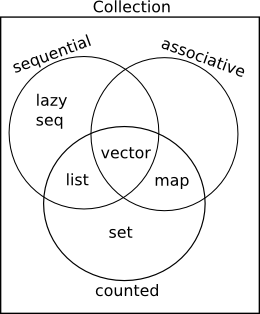• 数据结构
• Collection 和 Sequence
• Sequence & List
• Vector
• Map
• Set
• 常用函数

## 数据结构

### Collection 和 Sequence

Clojure 常用的数据结构有 List, Map, Vector, Set.
他们都属于 Collection, 之间的关系大致是这样:属于 Clojure 当中实现的数据结构都是 Collection. 编码当中会遇到 Host 平台的数据类型, 不属于 Collection.

实现了 Collection 的接口的数据结构都支持这些函数: =, count, conj, empty, seq.
实现了 Sequence 的接口的数据结构支持这些函数: first, rest, next.

关于数据结构的细节推荐阅读 Collections and Sequences in Clojure.

Clojure 中的数据结构被设计成不可变的, 用来配合函数式编程. 这和主流编程语言存在区别.

### Sequence & List

Clojure 中的 List 是单链表. 适合从头部访问, 而不适合随机访问. Clojure 采用 Lisp 语法来定义 List:

'(1 2 3 4); (1 2 3 4)(list? '(1 2 3 4)); true

这个语法实际上等同于 quote:

(quote (1 2 3 4)); (1 2 3 4)

或者通过调用的写法可以创建 List:

(list 1 2 3 4); (1 2 3 4)

Sequence 是一种抽象的数据结构, List 本身是一种 Sequence, 同时 Sequence 本身可以表示惰性的列表.

(type (range)); cljs.core/Range(seq? (range)); true

可以用 seq 函数来创建 Sequence, 不过可以粗略地把它被 List 混在一起理解和使用:

(seq [1 2 3]); (1 2 3)

常用的操作:

(first (list 1 2 3 4)); 1(rest (list 1 2 3 4)); (2 3 4)(cons 1 '(2 3 4 5 6)); (1 2 3 4 5 6)

### Vector

Vector 支持随机访问, 通过 [] 语法可以快速定义:

[1 2 3 4]; [1 2 3 4](vector? []); true

也支持是用 vecvector 函数通过调用的方式定义:

(vector 1 2 3 4); [1 2 3 4](vec '(1 2 3 4)); [1 2 3 4]

常用的操作比如:

(get [:a :b :c :d] 1); :b(nth [:a :b :c :d] 1); :b(peek [1 2 3 4]); 4(pop [1 2 3]); [1 2](conj [1 2 3] 4); [1 2 3 4](subvec [1 2 3] 1 2) ; 截取向量; 

### Map

Map 是关联列表, 可以通过 {} 语法定义 Map, 其中 , 等同于空白. 一般键和值的类型没有具体限制:

{:a "a", "b" 3, 4 []}; {:a "a", "b" 3, 4 []}(map? {}); true

一般使用当中会用 Keyword 来作为键:

{:name "Clojure", :born 2007}

除了通过语法, 也可以用 hash-map 等函数通过调用的方式创建:

(hash-map 1 2 3 4); {1 2, 3 4}(zipmap [:a :b :c] [1 2 3]); {:a 1, :b 2, :c 3}

常用的操作有:

(:a {:a 1}); 1(assoc {:a 1, :b 2} :c 3); {:a 1, :b 2, :c 3}(dissoc {:a 1} :b 2); {:a 1}(contains? {:a 1} :b); false(merge {:a 1} {:b 2}); {:a 1, :b 2}(update {:a 1} :a inc); {:a 2}

### Set

集合, 相同的元素之允许出现一次, 可以用 #{} 语法创建:

#{1 2 3}; #{1 2 3}(set? #{}); true

或者通过调用的方式创建:

(hash-set 1 2 3); #{1 2 3}(set [1 2 3]); #{1 2 3}

常用的操作有:

(conj #{1 2 3} 4); #{1 2 3 4}(disj #{1 2 3} 3); #{1 2}(contains? #{1 2 3} 4); false

此外 clojure.set 提供了其他一些集合的常用函数.

### 常用函数

推荐去 Cheatsheet 了解有哪些语言本身提供的函数.

• into

除了前面提到的函数, 还可以通过 into 来做强制数据结构转换:

(into [] '(1 2 3)); [1 2 3](into #{} '(1 2 3)); #{1 2 3}(into '() [1 2 3]); (3 2 1)(into {} [[:a 1] [:b 2]]); {:a 1, :b 2}
• first

first 取出 Sequence 的第一个元素, 但是对于 Map 来说能获取键值对:

(first [1 2 3]); 1(first '(1 2 3)); 1(first #{1 2 3}); 1(first {:a 1 :b 2}); [:a 1]
• map

注意 map 函数返回的是 Sequence, 并不是和原始的数据类型一致, 而且 reduce 等函数也是这样的:

(map inc [1 2 3]); (2 3 4)(map inc '(1 2 3)); (2 3 4)(map inc #{1 2 3}); (2 3 4)(map identity {:a 1 :b 2}); ([:a 1] [:b 2])

具体到 Vector 的情况下, 可使用 mapv 来得到 Vector 类型的结果, 或者用 into 强行转换:

(mapv identity {:a 1 :b 2}); [[:a 1] [:b 2]](into [] (map identity {:a 1 :b 2})); [[:a 1] [:b 2]]
• peek, popconj

由于 Sequence 和 Vector 存在区别, 有些函数虽然通用, 但是效果并不一样:

(peek [1 2 3]); 3(peek '(1 2 3)); 1(pop '(1 2 3)); (2 3)(pop [1 2 3]); [1 2](conj [1 2 3] 4); [1 2 3 4](conj '(1 2 3) 4); (4 1 2 3)
• count
(count '(1 2 3)); 3(count [1 2 3]); 3(count "123"); 3(count #{1 2 3}); 3(count {:a 1 :b 2 :c 3}); 3

对于长度为 0 可以用 empty? 函数来判断:

(empty? []); true(empty? {}); true(empty? #{}); true(empty? '()); true
• assoc-inupdate-in

对于嵌套比较深的数据结构, 会用到这些函数, 主要是 Vector, Map 或者混用两种类型:

(get-in {:a [1 2 3]} [:a 0]); 1(assoc-in {:a [1 2 3]} [:a 0] 4); {:a [4 2 3]}(update-in {:a [1 2 3]} [:a 0] inc); {:a [2 2 3]}

更多的函数还是建议阅读 Cheatsheet